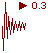Expert Modelling: Hands-on help from Rocscience Engineers. Register Here

## Search Results

In the Seismic Load dialog, you may define seismic coefficients for the Horizontal and / or Vertical directions. The Seismic Coefficients are dimensionless coefficients which represent the (maximum) earthquake acceleration as a fraction of the acceleration due to gravity. Typical values are in the range of 0.1 to 0.3.

Seismic Force

If seismic coefficients are defined, a seismic force will be applied to each slice as follows:

Seismic Force = Seismic Coefficient * Slice Weight

= Seismic Coefficient * area of slice * Unit Weight of slice material

Note that the acceleration due to gravity (g = 9.81 m/s2 for metric units) is already incorporated into the material Unit Weight entered in the Define Material Properties dialog (remember that the dimensions of Unit Weight are kN / m3), and therefore does not explicitly appear in the above equation.

The seismic force is applied through the centroid of each slice.

Horizontal Seismic Coefficient

The HORIZONTAL seismic coefficient is always POSITIVE, and represents a horizontal seismic force directed OUT OF the slope (i.e. in the direction of failure).

Since the HORIZONTAL seismic force in Slide2 is always directed OUT OF the slope, a HORIZONTAL seismic coefficient should always DECREASE the safety factor, for a given slip surface, compared to the same model with NO seismic load applied.

Vertical Seismic Coefficient

The VERTICAL seismic coefficient may be either POSITIVE or NEGATIVE.

• A POSITIVE vertical seismic coefficient represents a vertical seismic force directed DOWNWARDS.
• A NEGATIVE vertical seismic coefficient represents a vertical seismic force directed UPWARDS.

The effect of a VERTICAL seismic force is less obvious. A VERTICAL seismic coefficient may either DECREASE or INCREASE the safety factor, since the vertical seismic force affects the normal stress, and hence the shear strength, at the base of each slice. Experiment with different POSITIVE and NEGATIVE values of the VERTICAL seismic coefficient, and observe the effect on safety factor.

TIP: you can use the Sensitivity Analysis feature of Slide2 to easily determine the effect of the vertical seismic coefficient, over any desired range of values.

Excess Pore Pressure

The Excess Pore Pressure option in the Seismic Load dialog, will only be available if the "Calculate Excess Pore Pressure (B-bar method)" checkbox is selected in the Groundwater tab of the Project Settings dialog.

If you select the "Load creates excess pore pressure" checkbox, then a VERTICAL seismic load will generate excess pore pressure for all materials with B-bar > 0. This only applies to the VERTICAL seismic coefficient. It does NOT apply to the HORIZONTAL seismic coefficient. For more information see the Excess Pore Pressure topic.